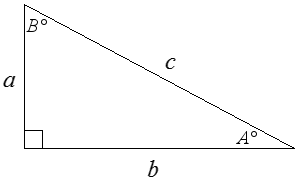OML-IZ Search

## TangentsWith reference to the diagram above, calculate and enter the answers. Round your answers to the nearest hundredth. You may use the TAB key to move to the next question. When you are done, click Submit.

1. B = 39, b = 4.7, a =
2. B = 76, a = 2.2, b =
3. B = 24, a = 2.7, b =
4. a = 3.1, b = 4.7, A =
5. A = 51, b = 6.9, a =
6. a = 5.7, b = 8.8, A =
7. B = 81, a = 8.6, b =
8. a = 5.3, b = 8.4, A =
9. A = 53, a = 10, b =
10. B = 44, b = 4.8, a =

We hope that the free math worksheets have been helpful. We encourage parents and teachers to adjust the worksheets according to the needs of the child. For more difficult questions, the child may be encouraged to work out the problem on a piece of paper before entering the solution. We hope that the kids will also love the fun stuff and puzzles.